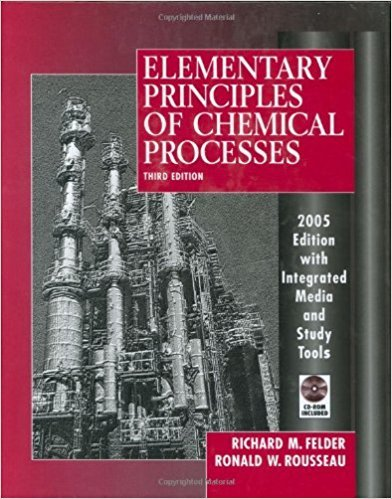×
Get Full Access to Elementary Principles Of Chemical Processes - 3 Edition - Chapter 9 - Problem 9.25
Get Full Access to Elementary Principles Of Chemical Processes - 3 Edition - Chapter 9 - Problem 9.25

×

# A gas mixture containing 85 mole% methane and the balanceISBN: 9780471687573 143

## Solution for problem 9.25 Chapter 9

Elementary Principles of Chemical Processes | 3rd Edition

• Textbook Solutions
• 2901 Step-by-step solutions solved by professors and subject experts
• Get 24/7 help from StudySoup virtual teaching assistantsElementary Principles of Chemical Processes | 3rd Edition

4 5 1 356 Reviews
29
4
Problem 9.25

A gas mixture containing 85 mole% methane and the balance oxygen is to be charged into an evacuated well-insulated lO-liter reaction vessel at 25C and 200 kPa. An electrical coil in the reactor, which delivers heat at a rate of 100 watts, will be turned on for 85 seconds and then turned off. Formaldehyde will be produced in the reaction CH4 + O2 ----. HCHO + H20 The reaction products will be cooled and discharged from the reactor. (a) Calculate the maximum pressure that the reactor is likelv to have to withstand, assuming that there are no side reactions. If you were ordering the re~ctor. why would you specify an even greater pressure in your order? (Give several reasons.) (b) Why would heat be added to the feed mixture rather than running the reactor adiabatically? (c) Suppose the reaction is run as planned, the reaction products are analyzed chromatographically, 486 Chapter 9 Balances on Reactive Processes and some CO2 is found. Where did it come from? If you had taken it into account, would Your calculated pressure in part (a) have been larger, smaller, or can't you tell without doing the de tailed calculations?

Step-by-Step Solution:

Problem 9.25A gas mixture containing 85 mole% methane and the balance oxygen is to be charged into anevacuated well-insulated 10-liter reaction vessel at 25°C and 200 kPa. An electrical coil in thereactor, which delivers heat at a rate of 100 watts, will be turned on for 85 seconds and thenturned off. Formaldehyde will be produced in the reaction CH4(g) + O2(g) ----. HCHO(g) +H20(g) The reaction products will be cooled and discharged from the reactor.(a) Calculate the maximum pressure that the reactor is likely to have to withstand, assuming thatthere are no side reactions. If you were ordering the reactor, why would you specify an evengreater pressure in your order (Give several reasons.)(b) Why would heat be added to the feed mixture rather than running the reactor adiabatically(c) Suppose the reaction is run as planned, the reaction products are analyzedchromatographically, 486 Chapter 9 Balances on Reactive Processes and some CO2 is found.Where did it come from If you had taken it into account, would Your calculated pressure in part(a) have been larger, smaller, or can't you tell without doing the detailed calculations Step-by-step solution Step 1 of 9 ^The flowchart for a process to produce formaldehyde is shown below.The reaction involved is shown below. CH 4O 2HCHO+H O 2(a)Calculate nF the number of moles charged to the reactor using the ideal gas law. PV nF RT\n 3 (200 kPa)( 1000 Pa )(10.0 L) 1 m = 1 kPa ( 1000 L) (8.314 m .Pa) (298 K) mol.KDetermine the initial number of moles of each compound. mol CH4 (0.85 mol ) (0.807 mol) = (0.15 mol O 20.807 mol) =

Step 2 of 9

Step 3 of 9

##### ISBN: 9780471687573

Unlock Textbook Solution